Principal ideal domain

This article defines a property of integral domains, viz., a property that, given any integral domain, is either true or false for that.
The corrresponding general property for commutative unital rings is: principal ideal ring
View other properties of integral domains | View all properties of commutative unital rings
VIEW RELATED: Commutative unital ring property implications | Commutative unital ring property non-implications |Commutative unital ring metaproperty satisfactions | Commutative unital ring metaproperty dissatisfactions | Commutative unital ring property satisfactions | Commutative unital ring property dissatisfactions

Definition

Below are some equivalent definitions of principal ideal domain (also known as PID) if it is an integral domain and a principal ideal ring. Explicitly, it must satisfy the following equivalent conditions:

No. Shorthand A commutative unital ring is termed a principal ideal domain if ... A commutative unital ring$R$ is termed a principal ideal domain if ...
1 every ideal principal it is an integral domain and every ideal in it is principal$R$ is an integral domain (i.e.,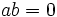$ab = 0$ implies$a = 0$ or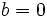$b = 0$) and for every ideal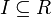$I \subseteq R$, there exists$x \in I$ such that$I = (x)$, i.e.,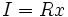$I = Rx$.
2 every prime ideal principal it is an integral domain and every prime ideal in it is principal$R$ is an integral domain (i.e.,$ab = 0$ implies$a = 0$ or$b = 0$) and for every prime ideal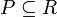$P \subseteq R$, there exists$x \in P$ such that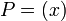$P = (x)$, i.e.,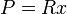$P = Rx$.
3 Dedekind-Hasse norm it admits a Dedekind-Hasse norm there is a Dedekind-Hasse norm on$R$.

Relation with other properties

Expression as a conjunction of other properties

First component of conjunction Second component of conjunction Proof
principal ideal ring integral domain by definition
Noetherian domain Bezout domain Noetherian and Bezout iff principal ideal
unique factorization domain Bezout domain Unique factorization and Bezout iff principal ideal
unique factorization domain Dedekind domain (see next)
unique factorization domain one-dimensional domain Unique factorization and one-dimensional iff principal ideal

Stronger properties

Property Meaning Proof of implication Proof of strictness (reverse implication failure) Intermediate notions
Euclidean domain has a Euclidean norm Euclidean implies PID PID not implies Euclidean click here
Polynomial ring over a field$k[x]$ for a field$k$ (via Euclidean domain) (via Euclidean domain) click here

Weaker properties

Property Meaning Proof of implication Proof of strictness (reverse implication failure) Intermediate notions
Dedekind domain PID implies Dedekind Dedekind not implies PID click here
Bezout domain integral domain where every finitely generated ideal is principal PID implies Bezout Bezout not implies PID click here
Noetherian domain integral domain that's also a Noetherian ring PID implies Noetherian Noetherian not implies principal ideal click here
unique factorization domain integral domain with unique factorization PID implies UFD UFD not implies PID click here
normal domain integral domain that is integrally closed in its field of fractions (via UFD) (via UFD) click here
Noetherian normal domain Noetherian ring and normal domain click here
One-dimensional domain integral domain in which every nonzero prime ideal is maximal PID implies one-dimensional One-dimensional domain not implies PID click here
One-dimensional Noetherian domain one-dimensional and Noetherian click here
principal ideal ring every ideal is principal, not necessarily a domain click here
catenary ring any two saturated chains of primes with the same starting and ending point have the same length click here

Metaproperties

Metaproperty name Satisfied? Proof Statement with symbols
polynomial-closed property of commutative unital rings No follows from Polynomial ring over a ring is a PID iff the ring is a field It is possible to have a principal ideal domain$R$ such that the polynomial ring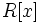$R[x]$ is not a principal ideal domain. In fact,$R[x]$ is a principal ideal domain if and only if$R$ is a field, so for instance$R = \mathbb{Z}$ or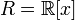$R = \mathbb{R}[x]$ give counterexamples.

Closure under taking localizations

This property of integral domains is closed under taking localizations: the localization at a multiplicatively closed subset of a commutative unital ring with this property, also has this property. In particular, the localization at a prime ideal, and the localization at a maximal ideal, have the property.
View other localization-closed properties of integral domains | View other localization-closed properties of commutative unital rings

If we localize a principal ideal domain at any multiplicatively closed subset that does not contain zero, and in particular if we localize relative to a prime ideal, we continue to get a principal ideal domain. The reason is, roughly, that any ideal in the localization is generated by the element in the contraction that generates it.

Closure under quotients by prime ideals

This property of integral domains is prime-quotient-closed: the quotient of any integral domain satisfying this property by a prime ideal also satisfies the property. Note that we need the ideal to be prime for the quotient to also be an integral domain.
View other prime-quotient-closed properties of integral domains

The quotient of a principal ideal domain by any prime ideal is again a principal ideal domain. In fact, the quotient is either equal to the original domain (in case the prime ideal is zero) or is a field (in case the prime ideal is maximal. This is because in a principal ideal domain, every nonzero prime ideal is maximal.

However, it is true in slightly greater generality that the quotient of a principal ideal ring by any ideal is again a principal ideal ring. For full proof, refer: Principal ideal ring is quotient-closed

Module theory

Further information: structure theory of modules over PIDs

Any finitely generated module$M$ over a PID$R$ can be expressed as follows: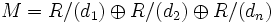$M = R/(d_1) \oplus R/(d_2) \oplus R/(d_n)$

where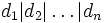$d_1|d_2|\ldots|d_n$. Some of the$d_n$ could be zero.

The$d_i$ are unique upto units; the principal ideals they generate are unique.

There is another equivalent formulation:$M = R/(p_1^{k_1}) \oplus R/(p_2^{k_2}) \oplus R/(p_r^{k_r})$

Where all the$p_i$ are prime.

Thus, a finitely generated module over a PID is projective if and only if it is free.